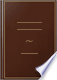Αναζήτηση Εικόνες Χάρτες Play YouTube Ειδήσεις Gmail Drive Περισσότερα »
Είσοδος
 Βιβλία Βιβλία 21 - 30 από 180 για The dividend is the number to be divided. The divisor is the number by which we divide..The dividend is the number to be divided. The divisor is the number by which we divide.A Complete Arithmetic: Uniting Mental and Written Exercises in a Natural ... - Σελίδα 27
των Emerson Elbridge White - 1870 - 340 σελίδες
Πλήρης προβολή - Σχετικά με αυτό το βιβλίο## The national arithmetic on the inductive system: combining the analytic and ...

Benjamin Greenleaf - 1858 - 444 σελίδες
...Neptune is 30 times as far. How far is Neptune from the Sun ? Ans. 2,850,000,000 miles. DIVISION. 66. Division is the process of finding how many times one number is contained in another ; or the process of separating a number into a proposed number of equal parts. In division there are three...## The National Arithmetic on the Inductive System: Combining the Analytic and ...

Benjamin Greenleaf - 1858 - 444 σελίδες
...Neptune is 30 times as far. How far is Neptune from the Sun ? Ans. 2,850,000,000 miles. DIVISION. 66. DIVISION is the process of finding how many times one number is contained in another ; or the process of separating a number into a proposed number of equal parts. In division there are three...## Greenleaf's Intellectual Arithmetic

Benjamin Greenleaf - 1858 - 154 σελίδες
...tens 1 ten time, and 3 tons remainder ; 6 in 3 tens, or 30, 5 tunes ; answer, 15. 3. Divide 96 by 8. * DIVISION is the process of finding how many times one number is contained in another, or the process of separating a number into any proposed number of equal parts. Tho number to be divided...## A Mental Arithmetic, Upon the Inductive Plan: With Easy Exercises for the ...

Benjamin Greenleaf - 1858 - 72 σελίδες
...earth ? LESSON XI. DIVISION. 1. In 24 how many times 4 ? How many times 6 ? How many times 8 ? 2. What is the process of finding how many times one number is contained in another called ? ANS. Division. 8. What is the number to be divided called ? ANS. The Dividend. 4. What is...## The Progressive Intellectual Arithmetic, on the Inductive Plan: Being a ...

Horatio Nelson Robinson - 1859
...miles an hour, and the other at the rate of 11 miles an hour, how many hours before they will meet ? Division is the process of finding how many times one number is contained in another ; and the result is called the Quotient. The sign of division is written thus, -;-, and, placed between...## The Progressive Practical Arithmetic: Containing the Theory of Numbers, in ...

Horatio Nelson Robinson - 1859 - 336 σελίδες
...in 64 ? 14. How many times 9 in 36? in 63? in 81 ? 15. How many times 7 in 49 ? in 70 ? in 84 ? 72. Division is the process of finding how many times one number is contained in another. 73. The Dividend is the number to be divided. 74. The Divisor is the number to divide by. 75. The Quotient...## A Mental Arithmetic, Upon the Inductive Plan

Benjamin Greenleaf - 1860 - 156 σελίδες
...8. NOTE. 6 in 9 tens 1 ton time, and 3 tens remainder ; 6 in S tens, or 30, 5 times ; answer, 15. * DIVISION is the process of finding how many times one number is contained in another, or the process of separating a number into any proposed number of equal parts. The number to be divided...## The Progressive Intellectual Arithmetic on the Inductive Plan: Being a ...

Horatio Nelson Robinson - 1860 - 176 σελίδες
...an hour, and the other at the rate of 11 miles an hour, how many hours before they will meet ? 23. Division is the process of finding how many times one number is contained in another; and the result is called the Quotient. The sign of division is written thus, +, and, placed between...## The Progressive Higher Arithmetic: For Schools, Academies, and Mercantile ...

Horatio Nelson Robinson - 1860 - 432 σελίδες
...three dollar pieces ; what was the total value of this gold coin ? Ans. \$29,507,732. DIVISION. 1O3. Division is the process of finding how many times one number is contained in another. The Dividend is the number to he divided. The Divisor is the number to divide by. lOS. The Quotient...## Introduction to the National Arithmetic, on the Inductive System: Combining ...

Benjamin Greenleaf - 1861 - 324 σελίδες
...many times 11 in 22 ? In 55? In 77? In 88? In 110? In 132? ART. 46i The pupil will now perceive that DIVISION is the process of finding how many times one number is contained in another. In division there are three principal terms: the Dividend the Divisor, and the Quotient, or answer....